Symbol
ProblemWhich line is parallel to $y=3x-12x$ $○$ $y=4x-1$ $○$ $y=-3x+5$ $○$ $y=3x$ $○$ $y=-3x+6$
Algebra
SolutionQanda teacher - Sahilkhan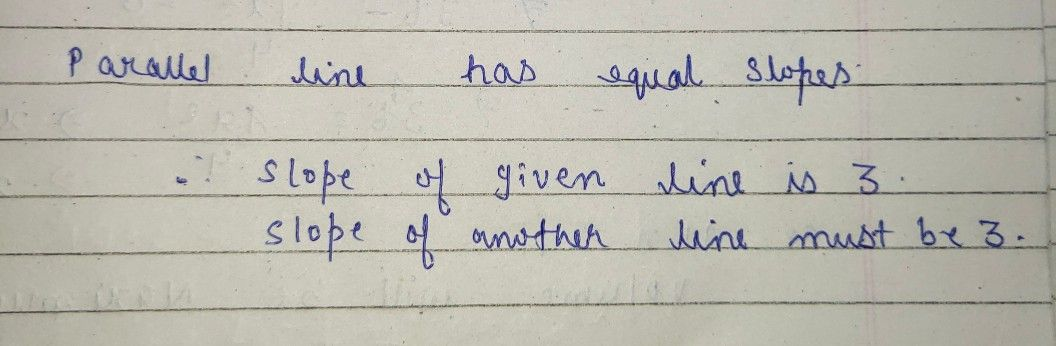so option C is the correct answerStudent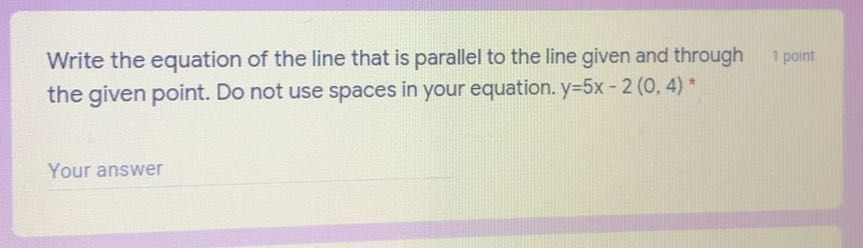Qanda teacher - Sahilkhan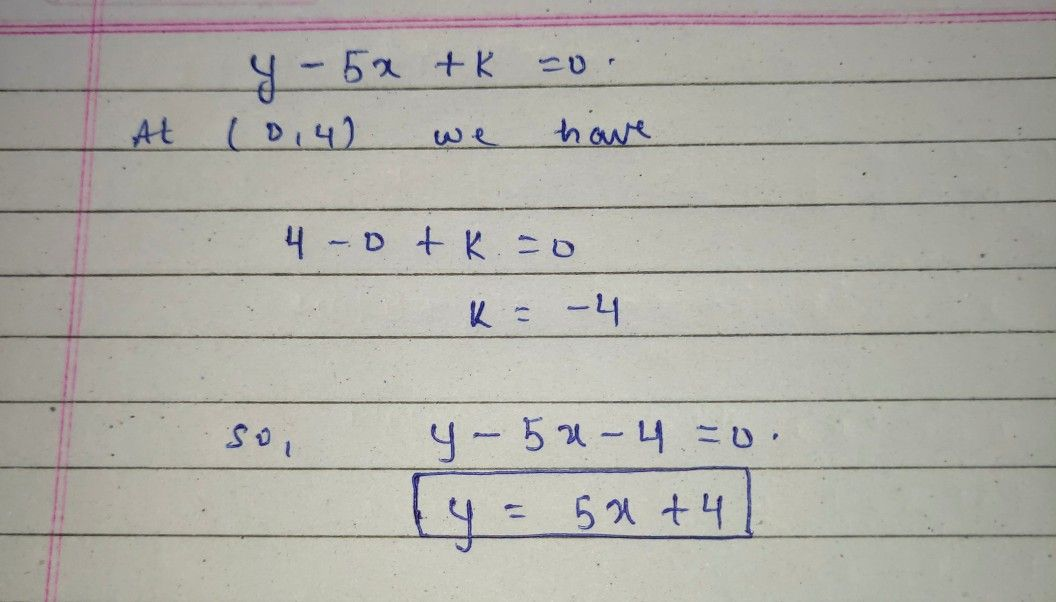Student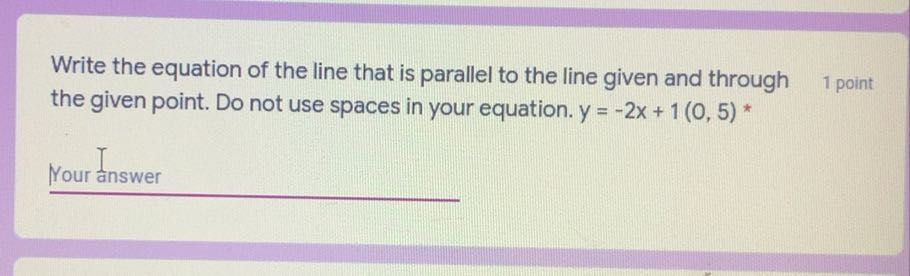Qanda teacher - Sahilkhan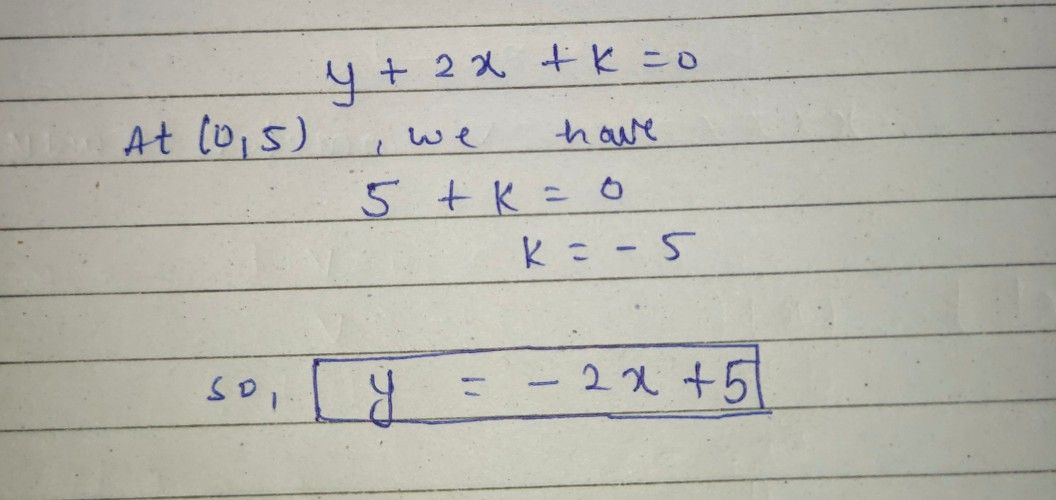ask questions in' preferred match questions'
so that I can get coins
or better you to gift me some coinsStudent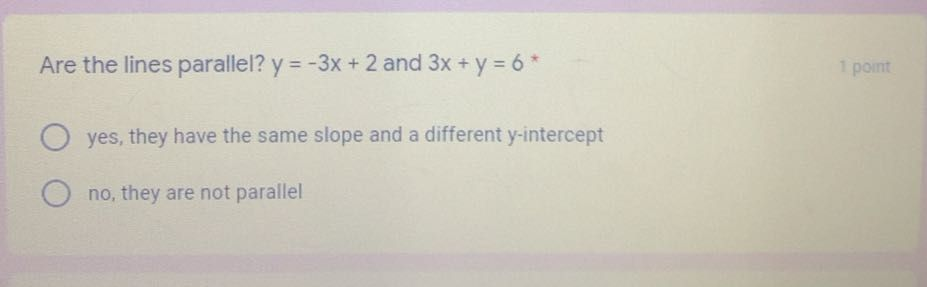Qanda teacher - Sahilkhan
yes they are parallel
because they have same slope and different y interceptStudent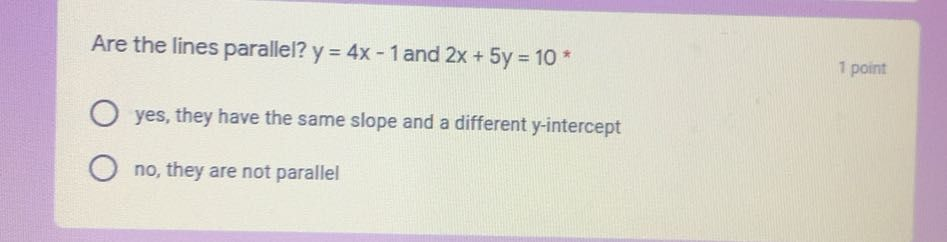Qanda teacher - Sahilkhan
no they are not parallel
they have different slopesStudent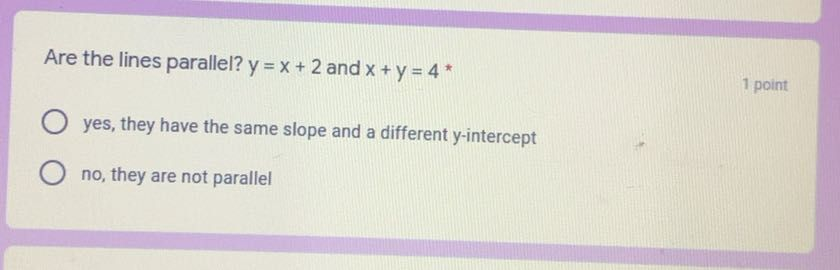Qanda teacher - Sahilkhan
no they are not parallel
rather they are perpendicularStudent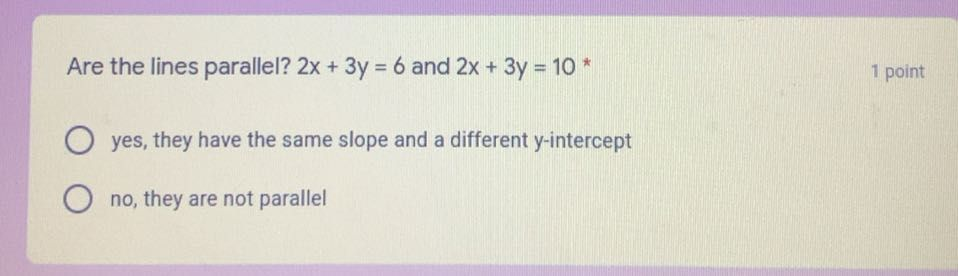Qanda teacher - Sahilkhan
yes they are parallelStudent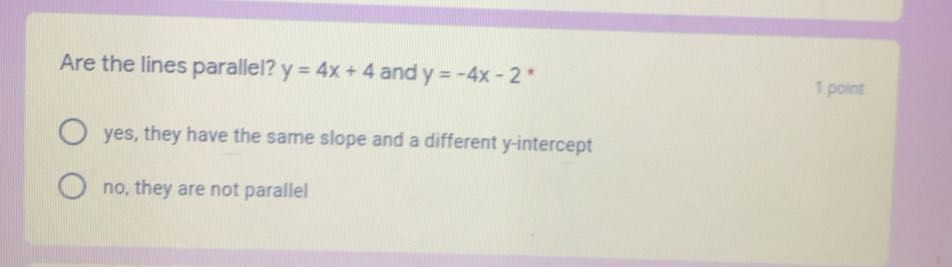Qanda teacher - Sahilkhan
no they are not parallelStudent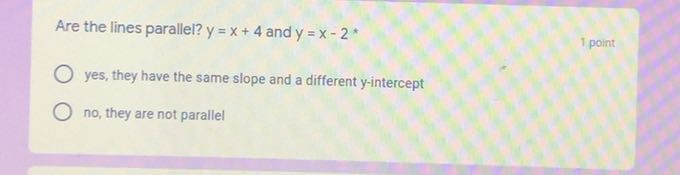Qanda teacher - Sahilkhan
yes they are parallelStudent
thank you so much ! I got a hundred!Qanda teacher - Sahilkhan
great ?
if possible gift some coins ?Student
will do !